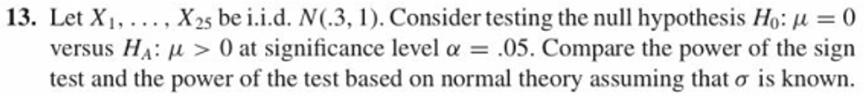# 13. Let x, . . . . . X.25 be i.id. N(3, l ). Consider testing the null hypothesis Ho: μ 0versus μ > 0 at significance level α-.05. Compare the power of the signtest and the power of the test based on normal theory assuming that σ is known.

Question
37 views

11.13 Attached. Thank you for answering my question!help_outlineImage Transcriptionclose13. Let x, . . . . . X.25 be i.id. N(3, l ). Consider testing the null hypothesis Ho: μ 0 versus μ > 0 at significance level α-.05. Compare the power of the sign test and the power of the test based on normal theory assuming that σ is known. fullscreen
check_circle

Step 1

The hypothesis:

The hypothesis of interest is: H0: μ = 0 vs. HA: μ > 0, at level of significance α = 0.05.

The sample size is n = 25. The random sample is: X1, X2, …, X25.

Step 2

Power for normality assumption:

Under the assumption of normal theory, X1, X2, …, X25 is from a normal distribution with parameters: mean 0.3 and standard deviation 1. The known standard deviation, σ is 1.

Thus, the sample mean for the sample of size 25 will have a normal distribution with mean 0.3 and standard deviation 1/√25 = 1/5 = 0.2.

The power of the test is the probability that the sample mean exceeds the critical value, that is, lies in the rejection region, when the alternative hypothesis, HA is true. Using the given information, the value of μ considered for the alternative HA: μ > 0 is μ = 0.3.

Now, zα = z0.05 is the upper α-point or the point of the standard normal variable, the probability that a value lies above which is α = 0.05, in this case.

The critical value for the test using sample mean is: C = μ0 + zασ/√n, where μ0 is the hypothesized mean, here, μ0 = 0; σ/√n = 0.2; z0.05 = 1.645.

Thus, the power of the test is calculated as follows:

Step 3

Power for sign test:

The normal distribution being symmetric, mean = median. So, here, median is μ.

For the sign test, denote S as the count or the number of observations among X1, X2, …, X25, that are greater than the hypothesized median, μ = 0.

It is known that half of all observations lie above the median and half lie below the median. If the null hypothesis, H0 is true, then the probability that an observation is greater than the hypothesized value 0 is 1/2 = 0.5. Here, sample size, n = 25.

Thus, according to the rules of a binomial model, S must follow the binomial distribution with parameters: number of trials 25 and probability of success...

### Want to see the full answer?

See Solution

#### Want to see this answer and more?

Solutions are written by subject experts who are available 24/7. Questions are typically answered within 1 hour.*

See Solution
*Response times may vary by subject and question.
Tagged in

### Statistics